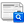## Journal of the European Optical Society - Rapid publications, Vol 6 (2011)

### Computation of Hopkins’ 3-circle integrals using Zernike expansions

A. J. E. M. Janssen

#### Abstract

The integrals occurring in optical diffraction theory under conditions of partial coherence have the form of an incomplete autocorrelation integral of the pupil function of the optical system. The incompleteness is embodied by a spatial coherence function of limited extent. In the case of circular optical systems and coherence functions supported by a disk, this gives rise to Hopkins’ 3-circle integrals. In this paper, a computation scheme for these integrals (initially with coherence functions that are constant on their disks) is proposed where the required integral is expressed semi-analytically in the Zernike expansion coefficients of the pupil function. To this end, the Zernike expansion coefficients of a shifted pupil function restricted to the coherence disk are expressed in terms of the pupil function’s Zernike expansion coefficients. Next, the required integral is expressed as an infinite series involving two sets of restricted Zernike coefficients using Parseval’s theorem for orthogonal series. The computation method is extended to the case of coherence functions that are not necessarily constant on their supporting disks by using a result on linearization of the product of two Zernike circle polynomials involving Wigner coefficients.

Full Text: PDF

#### Citation DetailsCite this article

#### References

M. Born, and E. Wolf, Principles of Optics (7th ed., Cambridge University Press, Cambridge, 1999).

H. H. Hopkins, "On the diffraction theory of optical images" P. R. Soc. A 217, 408-432 (1953).

H. H. Hopkins, "Diffraction theory of laser read-out systems for optical video discs" J. Opt. Soc. Am. 69, 4-24 (1979).

A. J. E. M. Janssen, "New analytic results for the Zernike circlepolynomials from a basic result in the Nijboer-Zernike diffraction theory" J. Eur. Opt. Soc.-Rapid 6, 11028 1-14 (2011).

M. Abramowitz, and I. A. Stegun, Handbook of Mathematical Functions (Dover Publications, New York, 1972).

B. R. A. Nijboer, The Diffraction Theory of Aberrations (Ph. D. thesis, University of Groningen, 1942).

A. J. E. M. Janssen, and P. Dirksen, "Computing Zernike polynomials of arbitrary degree using the discrete Fourier transform" J. Eur. Opt. Soc.-Rapid 2, 07012 1-3 (2007).

F. G. Tricomi, Vorlesungen über Orthogonalreihen (Springer-Verlag, Berlin, 1955).

J. J. M. Braat, P. Dirksen, A. J. E. M. Janssen, and A. S. van de Nes, "Extended Nijboer-Zernike representation of the vector field in the focal region of an aberrated high-aperture optical system" J. Opt. Soc. Am. A 20, 2281-2292 (2003).

W. J. Tango, "The circle polynomials of Zernike and their application in optics" Appl. Phys. 13, 327-332 (1977).

L. C. Biedenharn, and J. D. Louck, Angular Momentum in Quantum Physics (Addison-Wesley, Reading, Massachusetts, 1981).